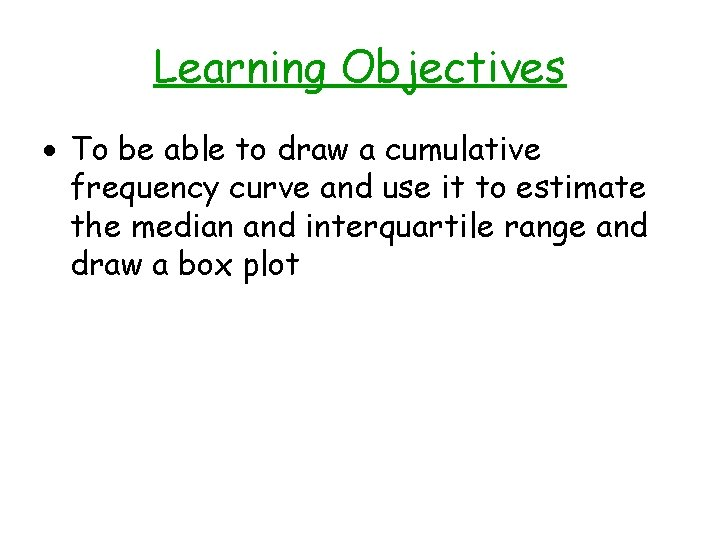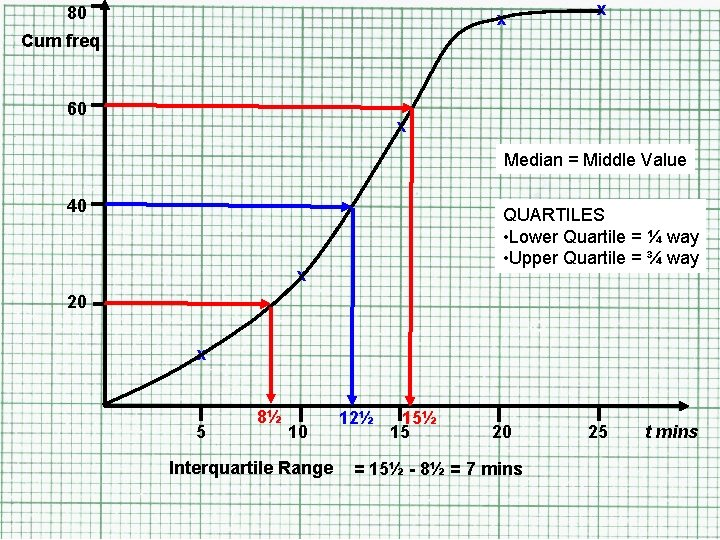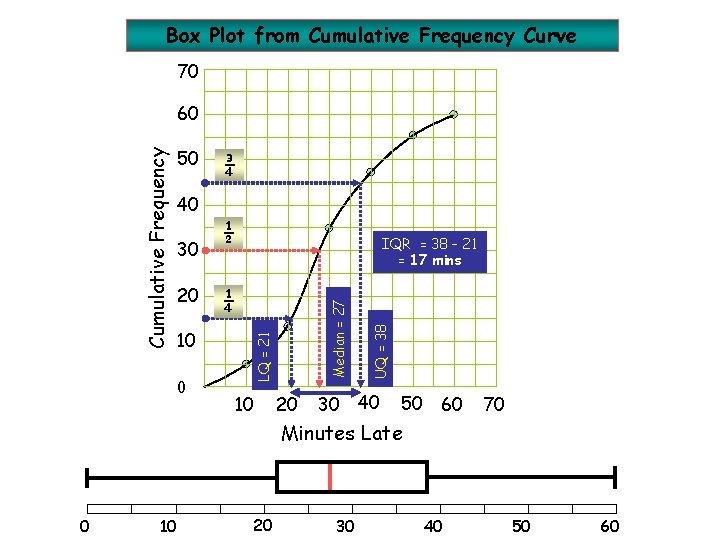# Cumulative Frequency and Box Plots Learning Objectives To

• Slides: 11Cumulative Frequency and Box PlotsLearning Objectives To be able to draw a cumulative frequency curve and use it to estimate the median and interquartile range and draw a box plotDoes anybody remember how to draw a Cumulative Frequency Diagram?Cumulative Frequency Curves • Cumulative Frequency = a running total of the data • Median = Half way up the Cumulative Frequency • Lower Quartile (LQ) = ¼ way up Cum Freq • Upper Quartile (UQ) = ¾ way up Cum Freq • Inter Quartile Range = UQ - LQBig Worked Example The table below shows the number of minutes students were late for their fun algebra lesson. (a) Draw a Cumulative Frequency Diagram of the data (b) Use it to find the Median, Lower Quartile, Upper Quartile, and Inter Quartile Range (c) Draw a Box-Plot assuming a minimum time of o minutes and a maximum of 25 minutes Time t (mins) Number of Students 0<t≤ 5 10 5 < t ≤ 10 16 10 < t ≤ 15 30 15 < t ≤ 20 22 20 < t ≤ 25 2 Cumulative Frequency80 x x Cum freq 60 x Median = Middle Value 40 QUARTILES • Lower Quartile = ¼ way • Upper Quartile = ¾ way x 20 x 5 8½ 10 Interquartile Range 12½ 15 20 = 15½ - 8½ = 7 mins 25 t minsDoes anybody have any idea what a Box Plot is?Box Plots • Box Plots (Box and Whisker Diagram) are another way of displaying data for comparison • To draw a Box Plot we need FIVE pieces of information: • – – – 1. 2. 3. 4. 5. The Minimum Value Lower Quartile (Q 1) The Median (Q 2) Upper Quartile (Q 3) Maximum Value They are drawn in the following way: 1 2 3 4 • BOX = The location of the middle 50% of the data • WHISKERS = How the data is spread overall 5Box Plot from Cumulative Frequency Curve 70 50 ¾ 40 10 0 ¼ 10 20 30 UQ = 38 20 IQR = 38 – 21 = 17 mins Median = 27 30 ½ LQ = 21 Cumulative Frequency 60 40 50 60 70 Minutes Late 0 10 20 30 40 50 60Box Plot Lowest value Lower Quartile 0 Highest value Median 5 10 Upper Quartile 15 20 25 t minsWork • Ex 7: 1 – Page 62 – Questions 5 and 6